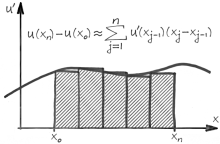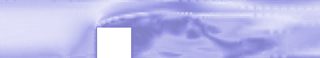## Body: ComputationMore on Body (computational examples)

## Soul: Mathematical AnalysisWelcome to the Body and Soul Project presenting a Reform Program for Applied Mathematics Education from introductory undergraduate to graduate level, supported by the Finite Element Center.

The project contains:

Main features of the program are:

• The program is based on a modern synthesis of Computation (Body) and Mathematical Analysis (Soul).
• The program may be described as Computational Mathematical Modeling CMM or the Calculus of the Computer Era.
• The program is both understandable and useful.
• The program presents a general powerful approach to CMM as a basis for the effective use of modern mathematical methods in mechanics, physics, chemistry and applied subjects.
• The program includes a large variety of educational material, which opens new possibilites of active learning.
• The program increases the motivation of the student by applying mathematical methods to interesting and important concrete problems already from the start.
• The program gives both theoretical and computational tools and builds confidence.
• The program contains most of the traditional material from basic courses in analysis and linear algebra, but also much more.